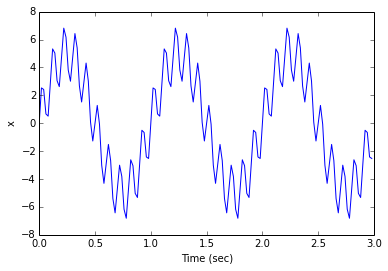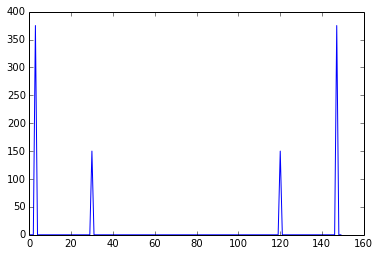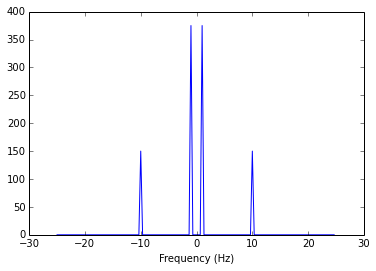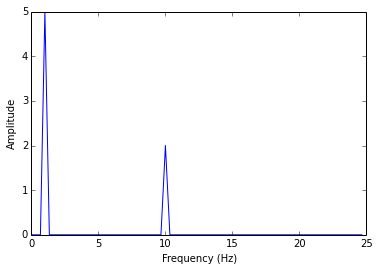# Working with Numpy FFT Results: Scaling and Folding¶

## Imports¶

In :
%matplotlib inline
import numpy as np
import matplotlib.pyplot as plt


## Create a Test Signal¶

$f_s$ is the sampling frequency, while $f$ is a base frequency for the signal content. We create a signal that contains components at a couple of multiples of this base frequency. Note the amplitudes here since we will be trying to extract those correctly from the FFT later.

In :
f_s = 50.0 # Hz
f = 1.0 # Hz
time = np.arange(0.0, 3.0, 1/f_s)
x = 5 * np.sin(2 * np.pi * f * time) + 2 * np.sin(10 * 2 * np.pi * f * time)

In :
plt.plot(time, x)
plt.xlabel("Time (sec)")
plt.ylabel("x")

Out:
<matplotlib.text.Text at 0x6e22b10>## Compute the FFT¶

The FFT and a matching vector of frequencies

In :
fft_x = np.fft.fft(x)
n = len(fft_x)
freq = np.fft.fftfreq(n, 1/f_s)
print n
print freq

150
[  0.           0.33333333   0.66666667   1.           1.33333333
1.66666667   2.           2.33333333   2.66666667   3.           3.33333333
3.66666667   4.           4.33333333   4.66666667   5.           5.33333333
5.66666667   6.           6.33333333   6.66666667   7.           7.33333333
7.66666667   8.           8.33333333   8.66666667   9.           9.33333333
9.66666667  10.          10.33333333  10.66666667  11.          11.33333333
11.66666667  12.          12.33333333  12.66666667  13.          13.33333333
13.66666667  14.          14.33333333  14.66666667  15.          15.33333333
15.66666667  16.          16.33333333  16.66666667  17.          17.33333333
17.66666667  18.          18.33333333  18.66666667  19.          19.33333333
19.66666667  20.          20.33333333  20.66666667  21.          21.33333333
21.66666667  22.          22.33333333  22.66666667  23.          23.33333333
23.66666667  24.          24.33333333  24.66666667 -25.         -24.66666667
-24.33333333 -24.         -23.66666667 -23.33333333 -23.         -22.66666667
-22.33333333 -22.         -21.66666667 -21.33333333 -21.         -20.66666667
-20.33333333 -20.         -19.66666667 -19.33333333 -19.         -18.66666667
-18.33333333 -18.         -17.66666667 -17.33333333 -17.         -16.66666667
-16.33333333 -16.         -15.66666667 -15.33333333 -15.         -14.66666667
-14.33333333 -14.         -13.66666667 -13.33333333 -13.         -12.66666667
-12.33333333 -12.         -11.66666667 -11.33333333 -11.         -10.66666667
-10.33333333 -10.          -9.66666667  -9.33333333  -9.          -8.66666667
-8.33333333  -8.          -7.66666667  -7.33333333  -7.          -6.66666667
-6.33333333  -6.          -5.66666667  -5.33333333  -5.          -4.66666667
-4.33333333  -4.          -3.66666667  -3.33333333  -3.          -2.66666667
-2.33333333  -2.          -1.66666667  -1.33333333  -1.          -0.66666667
-0.33333333]

In :
plt.plot(np.abs(fft_x))

Out:
[<matplotlib.lines.Line2D at 0x70986f0>]## Swap Half Spaces¶

Note that frequencies in the FFT and the freq vector go from zero to some larger positive number then from a large negative number back toward zero. We can swap that so that the DC component is in the center of the vector while maintaining a two-sided spectrum.

In :
fft_x_shifted = np.fft.fftshift(fft_x)
freq_shifted = np.fft.fftshift(freq)

In :
plt.plot(freq_shifted, np.abs(fft_x_shifted))
plt.xlabel("Frequency (Hz)")

Out:
<matplotlib.text.Text at 0x70a2b50>## Fold Negative Frequencies and Scale¶

It's actually more common to look at just the first half of the unshifted FFT and frequency vectors and fold all the amplitude information into the positive frequencies. Furthermore, to get ampltude right, we must normalize by the length of the original FFT. Note the factor of $2/n$ in the following which accomplishes both the folding and scaling.

In :
half_n = np.ceil(n/2.0)
fft_x_half = (2.0 / n) * fft_x[:half_n]
freq_half = freq[:half_n]

In :
plt.plot(freq_half, np.abs(fft_x_half))
plt.xlabel("Frequency (Hz)")
plt.ylabel("Amplitude")

Out:
<matplotlib.text.Text at 0x6edc150>Now the spectrum contains spikes at the correct amplitudes at only positive frequencies, which are the only ones with physicality.